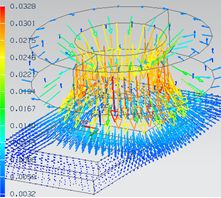Solutions

In this chapter the available solutions of the MAGNETICS for NX/Simcenter Solver are presented. Choose one solution to learn more about the theoretical background, the applicabilities and the solved results of the different solutions.

MagnetostaticThe magnetostatic solution describes the distribution of static magnetic fields due to permanent magnets and continuous currents.

Full Wave - TimeThe Full Wave Solver takes into account all terms of Maxwell's equations. Due to this the wavelength can be smaller than the analyzed part dimensions and even very high frequencies are allowed. In the time Domain results are the electric and magnetic field for every time step.

Magnetodynamic TimeThe magnetodynamic solution describes the distribution of magnetic fields and eddy currents due to excitation frequencies.

Electrodynamic Time

The electrodynamic solution describes the distribution of electric fields due to dynamically varying charges and/or levels of electric potential. This soluton allows time variations.

Full Wave - FrequencyThe Full Wave Solver takes into account all terms of Maxwell's equations. Due to this the wavelength can be smaller than the analyzed part dimensions and even very high frequencies are allowed. In the frequency domain Results are the electric and magnetic field due to excitation frequencies.

Magnetodynamic FrequencyThe magnetodynamic solution describes the distribution of magnetic fields and eddy currents due to excitation frequencies.

Electrodynamic Frequency

The electrodynamic solution describes the distribution of electric fields due to dynamically varying charges and/or levels of electric potential in frequency domain.

Electrokinetic (DC Conduction)The electrokinetic solution describes the distribution of static electric current in conductors.